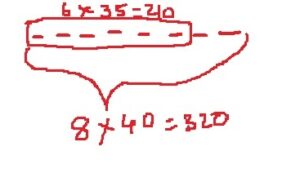# math problem solving skills – 10

The quantitative reasoning section tests your logical skills as well as your knowledge of math concepts.  To score high, you need to remember various formulas, theorems. Also you need to master critical problem-solving skills.

Today I am going to  take you through one problem -solving skill – Diagramming

Students with a non-math background usually.. do this

1. Read the complete math problem
2. Write equations.

Solving equations is time consuming.  You can make mistakes too..if your are not careful.

Students who are fairly proficient in quantitative reasoning..do this

1. Break the problem into parts. (they don’t read the whole question in one assay)
2. Analyze each part, and represent that part visually

Rarely do they write equations.

Then  they do mental calculations

This approach saves time…

Lets take this math problem

Observe the problem solving process…

## Method 1: Conventional approachAverage = sum of numbers/ number of items

Average of 8 terms = 40

So let the 8 terms be a,b,c,d,e,f,g,h

(a+b+c+d+e+f+g+h)/8 = 40

(a+b+c+d+e+f+g+h) = 40*8 = 320…..equation (i)

(a+b+c+d+e+f)/6 = 35

(a+b+c+d+e+f) =  35*6 = 210…..equation (ii)

Subtract both equations to get g+h

g+h= 320-210= 110

Average = 110/2 = 55

## Method 2: VisualizationLet’s visually represent the sum

Let’s take 8 terms as 8 dashes.The sum of the 8 terms = 8 x 40=320.  The sum of the first six terms is 6 x 35=210.

Subtract both sums to get the sum of the remaining terms = 320 – 210= 110

Hence average =110/2=55

The second method takes less time too.

So even if you have non math background, you can ace the math section by writing less equations and drawing more diagrams.

Feel free to contact me if you want to ace the math section by using simple strategies like this.